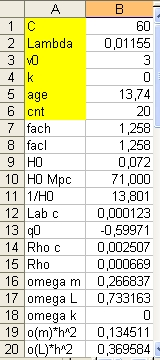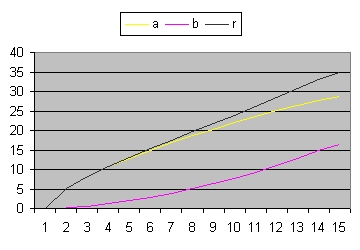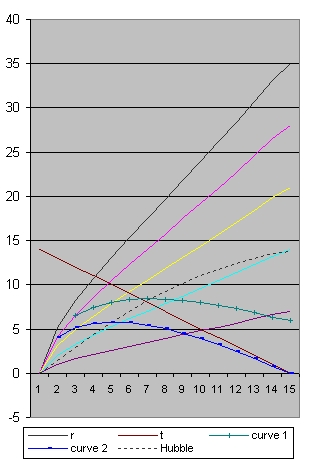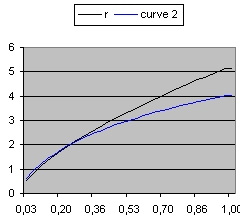### Answer question 3 - Lambda = 0,01155

This document shows the path of lightray starting close to the Big Bang which reaches the observer NOW.
The light ray is calculated using the Friedmann equation.
The parameters of the Friedmann equation reflect the most recent situation. For background information select this link: Background

• Figure 1 shows the parameters of the Excel program: "Friedmann's equation.xls"
The yellow parameters are input parameters.
The parameter "Lab C" is called "Lambda Critical" Which is defined as: 4 / (9 * C^2)
• Figure 1 A shows what is called the a and b curves. See Background information.
• Figure 1 B shows the path of a light ray.
• Figure 1 C shows a detail, close to the Big Bang. The earliest data is roughly 300000 years after the Big Bang.

In order to test the accuracy of the simulation use the CAMB Web Interface
Make the following modifications:
• Change Hubble Constant to 71.
• Change Omega(c) * h2 to 0.112
The result will be that Omega(m) * h2 = Omega(b) * h2 + Omega(c) * h2 = 0.1346
Now select GO
The results are: Age of the Universe = 13.707, Omega_M = 0.267011 and Omega_Lambda = 0.732989
Which is close to the results below:
Age of the Universe = 13.74, Omega_M = 0.266837 and Omega_Lambda = 0.733163Excel parameters Figure 1c = 60 Lambda = 0,01155 v0 = 3 Figure 1 Ac = 60 Lambda = 0,01155 v0 = 3
Figure 1 BFigure 1 C Detail Figure 1 C shows the detail of Figure 1 B in the range of 0 to 1 billion years. The black line represents the 100% distance line. The blue line shows the light ray starting from the Big Bang which reaches the Observer now.
The dashed line in Figure 1 B shows the Hubble sphere. This is radius where the recession velocity is equal to the speed of light.
Observe that this is the maximum distance from both the blue and the green line.
Back to
Friedmann's Equation Question 3